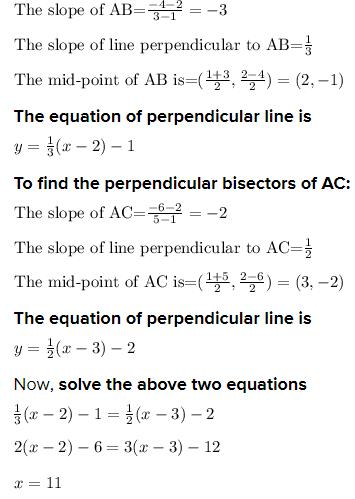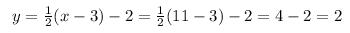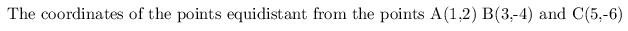Courses

# Test: Coordinate Geometry- 2

## 10 Questions MCQ Test General Aptitude for GATE 2020 | Test: Coordinate Geometry- 2

Description
This mock test of Test: Coordinate Geometry- 2 for Quant helps you for every Quant entrance exam. This contains 10 Multiple Choice Questions for Quant Test: Coordinate Geometry- 2 (mcq) to study with solutions a complete question bank. The solved questions answers in this Test: Coordinate Geometry- 2 quiz give you a good mix of easy questions and tough questions. Quant students definitely take this Test: Coordinate Geometry- 2 exercise for a better result in the exam. You can find other Test: Coordinate Geometry- 2 extra questions, long questions & short questions for Quant on EduRev as well by searching above.
QUESTION: 1

### Distance of a point from the origin: The distance of a point A(x, y) from the origin O(0, 0) is given by OA = √x2 + y2 Find the distance of the point A(4, -2) from the origin.

Solution:

OA = √4 - 02+(-2 - 0)2 = √16+4 = √20 = radic;4*5 = 2√5 units

QUESTION: 2

### Distance between two points : If (x1, y1) and B(x2, y2) be two points, then AB = √(x2 - x1)2 + (y2 - y1)2 Find the distance between the points A(-4, 7) and B(2, -5).

Solution:

AB = √(2+4)2 + (-5-7)2

= √62 + (-12)2

= √36+144 = √180

=√36*5 = 6√5 units.

QUESTION: 3

### The distance between the points A(b, 0) and B(0, a) is.

Solution:

AB = √(b-0)2-(0-a)2

= √b2+a2

= √a2+b2.

QUESTION: 4

If the distance of the point P(x, y) from A(a, 0) is a + x, then y2 = ?

Solution:

√(x-a)2+(y-0)2 = a + x

= (x-a)2+y2

= (a+x)2 => y2 = (x-a)2-(x-a)2-4ax => y2 = 4ax

QUESTION: 5

The distance between the points A(5, -7) and B(2, 3) is:

Solution:

AB2 = (2 - 5)2 + (3 + 7)2

=> (-3)2 + (10)2

=> 9 + 100 => √109

QUESTION: 6

Area of a triangle :

If A(x1,y1), B(x2,y2 and C(x3, y3) be three vertices of a ΔABC, then its area is given by:

Δ = 1/2 [x1(y2 - y3 + x2(y3 - y1) + x3(y1 - y2)]

Find the area of ΔABC whose vertices are A(9, -5), B(3, 7) and (-2, 4).

Solution:

Here, x1 = 9, x2 = 3, x3 = -2 and y1 = -5, y2 = 7, y3 = 4

= 1/2 [9(7-4) + 3(4+5) + (-2)(-5-7)]

= 1/2 [9(3) + 3(9) - 2(-12)]

= 1/2 [27 + 27 + 24]

= 1/2 

= 39 sq.units

QUESTION: 7

Find the area of ΔABC whose vertices are A(2, -5), B(4, 9) and (6, -1).

Solution:

Here, x1 = 2, x2 = 4, x3 = 6 and y1 = -5, y2 = 9, y3 = -1

= 1/2 [2(9+1) + 4(-1+5) + 6(-5-9)]

= 1/2 [2(10) + 4(4) + 6(-14)]

= 1/2 [20 + 16 - 84]

= 1/2 [-48]

= 24 sq.units

QUESTION: 8

The points A(0, 6), B(-5, 3) and C(3, 1) are the vertices of a triangle which is ?

Solution:

AB2= (-5 - 0)2 + (-3 - 0)2 = 16 + 9 = 25

BC2 = (3 + 5)2 + (1-3)2 = 82 + (-2)2 = 64 + 4 = 68

AC2 = (3 - 0)2 + (1 - 6)2 = 9 + 25 = 34.

AB = AC. ==> ΔABC is isosceles.

QUESTION: 9

The points A(-4, 0), B(1, -4), and C(5, 1) are the vertices of

Solution:

AB2 = (1 + 4)2 + (-4 - 0)2

= 25 + 16 = 41,

BC2 = (5 - 1)2 + (1 + 4)2 = 42 + 52

= 16 + 25 = 41

AC2 = (5 + 4)2 + (1 - 0)2

= 81 + 1 = 82

AB = BC and AB2 = BC2 = AC2

ΔABC is an isosceles right angled triangle

QUESTION: 10

Find the coordinates of the point equidistant from the points A(1, 2), B (3, –4) and C(5, –6).

Solution:

Given three points A(1,2) B(3,-4) and C(5,-6)

we have to find the coordinates of the point equidistant from the points.

The point that is equidistant from three points is called circumcenter which can be evaluated to find the perpendicular bisectors.

To find the perpendicular bisectors of AB:(11,2)